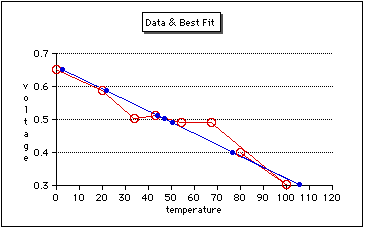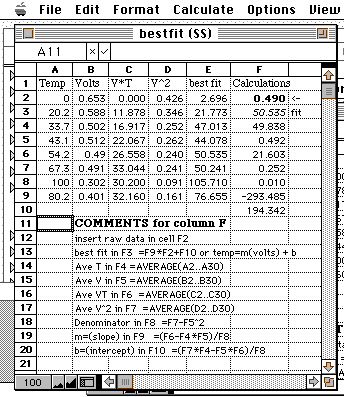# Hands-On-PhysicsHEAT & TEMPERATURE

## - Spreadsheets -

### Computing Best-Fits With a Spreadsheet

Reading a graph can be the largest source of error. Sometimes you want to determine the straight line that is a "best fit" to the data. Your data might have some "noise", random variations that cannot be reduced. For some reason, you expect your data to be on a straight line. You need to draw a straight line through the noisy data that is most likely to be right. This is determined from a statistical calculation called a "best fit straight line."Figure P10a
Best Fit Line

Here is a AppleWorks spreadsheet showing the formulas needed. You don't have to understand this; just copy this table, paste it directly into the upper left of a AppleWorks spreadsheet, and begin to use it. Enter your calibration data into cells A2...B9 and enter your raw data into cell F1. The best estimate of the temperature for that raw data will appear in cell F2. You can leave some calibration cells blank and you can add more calibration pairs, providing you copy columns C and D down to match your data.Figure P10b
Best Fit Calculation

As you can see there are two new columns, one for the product of voltage and temperature (V*T), and one for the square of the voltage (V^2). The averages of each of these four columns are computed in cells F4 through F7. Then these averages are used to compute the slope (a) and intercept (b) of the straight line fit to the data. Finally, these values are used in cell F2 to compute the best fit temperature from the raw data you put into cell F1. Column E has been used to calcuate best fit temperatures for all the voltage data in column B.

Excel has a FORCAST function which makes this process even simpler.

Previous Page || Up a Level || Index || Next Page